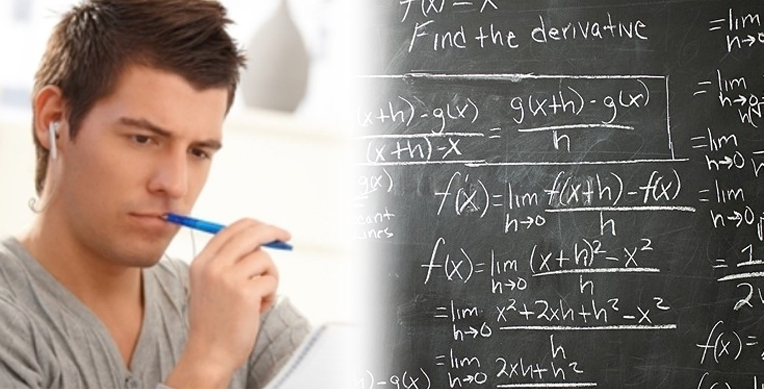Our Address: FZ LLC G 37 & G 34, Block 13, Al Sufouh Road Knowledge Park, Dubai PO Box : 501703

# The C TRAP in ALGEBRAIn order to solve system of equations involving two variables one knows from high school that two equations are required. This rule is not quite correct. The correct rule is you can solve a system of equation involving two variables if and only if you have two distinct linear equations.

Applying this rule incorrectly, particularly in Data sufficiency type questions, increases the chance of error.

For Example:

Q. What is the value of x?

1) x – 4y/7 = 8

2) 7x = 4y + 56

(A) Statement (1) ALONE is sufficient, but statement (2) alone is not sufficient.

(B) Statement (2) ALONE is sufficient, but statement (1) alone is not sufficient.

(C) BOTH statements TOGETHER are sufficient, but NEITHER statement ALONE is sufficient.

(D) EACH statement ALONE is sufficient.

(E) Statements (1) and (2) TOGETHER are NOT sufficient.

Approach:

If one solve this question hastily keeping the above rule in mind he will probably choose (C) right away. However, the correct answer is (E).

Solution:

Statement # 1 simplification:

x – 4y/7 = 8

7x – 4y = 56

Statement 1 is insufficient because one cannot solve a linear equation involving two variable with one equation.

Statement # 2 simplification:

7x = 4y + 56

7x – 4y = 56

This statement is also insufficient.

After simplification statement 1 and statement 2 are effectively the same equations; this mean that one cannot solve these two equations by using any method.

Example #2:

What is the value of x?

1) x – 6/y = 4

2) 2x + y = 16

Statement 1 and Statement 2 are clearly insufficient because of two variables in a single equation.

Now let’s solve taking both statements together:

Begin with the first equation.

x – 6/y = 4

Isolate x.

x = 6/y + 4

Substitute the value of x in the second equation

2(6/y + 4) +y = 16

After applying the distributive property of multiplication over parenthesis

12/y + 8 + y =16

Multiply both sides of the equation by y to clear the fraction.

12 + 8y + y2 = 16y

Transfer all terms to one side of the equation

y2 – 8y + 12 = 0

This is a quadratic equation with two solutions, but in Data sufficiency we need one unique answer for x and that is why both statements together are insufficient.